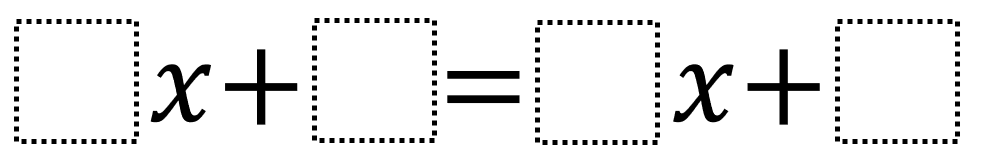# Linear Equations In One Variable 1

Directions: Using the digits 1 to 9 at most one time each, place a digit in each box to create two equations: one where x has a positive value and one where x has a negative value.### Hint

How does choosing the coefficient values affective whether the solution is positive or negative? How does choosing the constant values affective whether the solution is positive or negative?